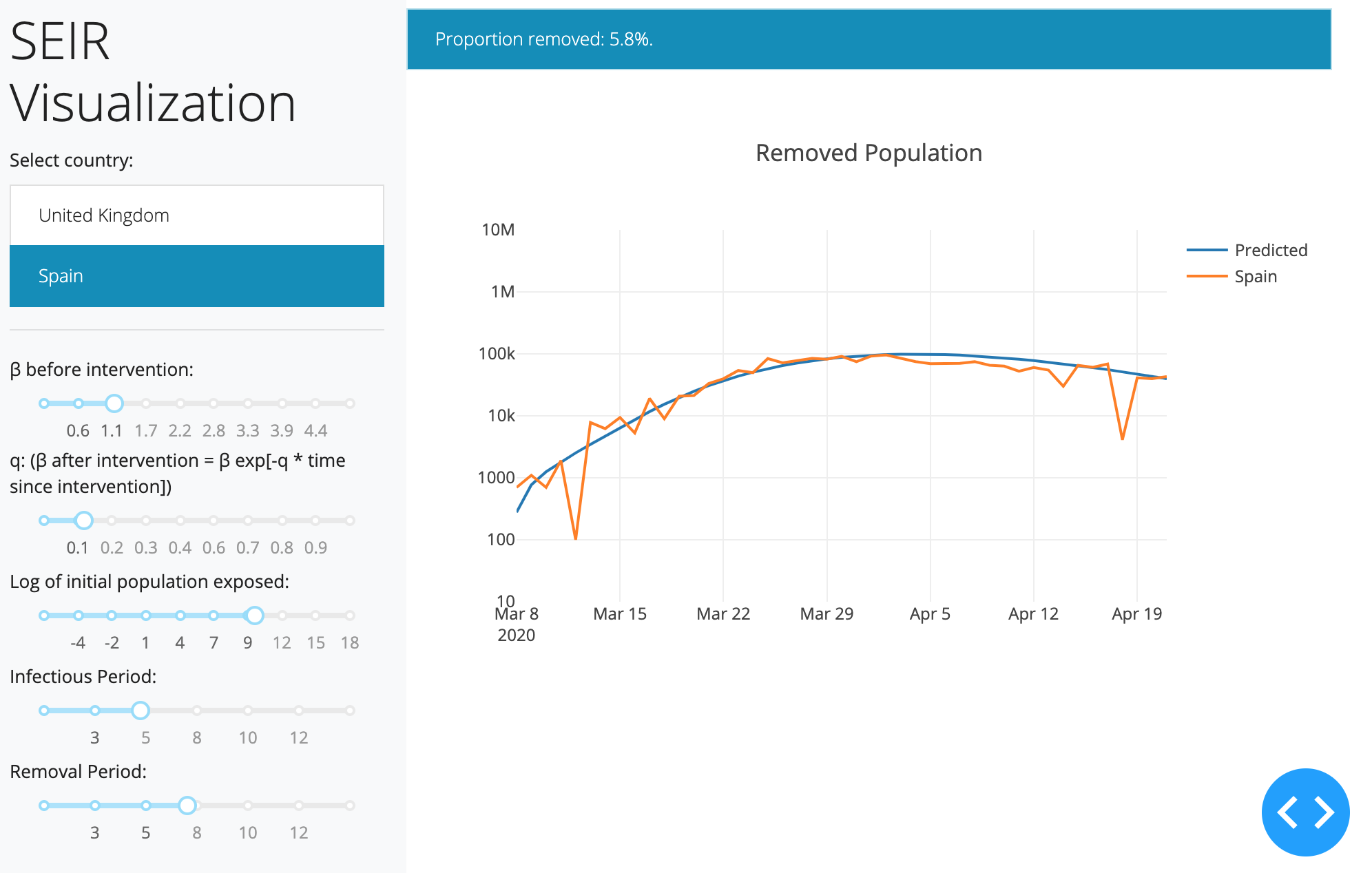I had a go at a few SEIR models, this is a rough diary of the process.

## Classical SEIR Model

The model is described on the Compartmental Models Wikipedia Page. Need to be careful about when the SEIR model starts though as none of the parameters explicitly control that aspect. I found it easier to truncate a bit of the data history before trying to minimize some kind of an error between predicted and actual cumulative “removed state” numbers. Since the paths are deterministic, this is quite easy to use. Rather than using Stan to optimize likelihoods, I found it easier to use black-box optimizers like PSO, GPO.

I wrote a quick-and-dirty Dash app to eyeball good initial starting values for the parameters (shown below; the values are most likely nonsensical at the moment). I’m also playing around with Imperial’s model.

Based on their assumptions, I made a few tweaks to mine (changed the beta parameter back to an exponential decay, used their IFR assumption). The infection-to-fatality ratio turned out to be a key assumption - setting this to 1% (following the Imperial model, based on Verity et al.) makes the results of this SEIR model quite close to the Imperial model results for one country I’m looking at, at the moment. The data for the app is based on a github repo, link is included in the code below.Quick & Dirty Dash App Code
Stan SEIR Implementation

## A Discrete Time SEIR Model

This was the first model that I tried. This is an implementation of the discrete time epidemiological (SEIR) model based on:

P. E. Lekone and B. F. Finkenstädt, “Statistical Inference in a Stochastic Epidemic SEIR Model with Control Intervention”, 2006.

I’ve made some changes to it, e.g. below, an intervention does not lead to an exponential decay of exposure probabilities - rather, the intervention considered here (a ‘lockdown’) just leads to lower exposure probabilities. If the population is large, the paths are very close to the model’s continuous time counterpart (the binomial variance is pretty small), so perhaps the stochastic treatment of the paths (and the resultant presence of so many hidden states) isn’t necessary here.

JAGS Code

I tried to use quite a few probabilistic programming languages:

• Stan: Didn’t work because integer parameters are not supported. Marginalizing the parameters would be very expensive I think due to the number of paths. Treating the parameters as real and making normal approximations with truncation was a nightmare (as linear combinations of parameters themselves had to be positive and I ran into precision issues).
• PyMC3: I’m a beginner with PyMC3 and my implementation was too inefficient. In the docs, the PyMC-devs suggest using theano scan instead of for-loops but I couldn’t figure out how parameter declarations worked in the backend. Code is still shown below if you’re interested.
• Tensorflow probability: I’m not used to the API and couldn’t find a Gibbs sampler.
• JAGS: Implementation was very simple and sampling works like a charm. Code above.
PyMC3 Naive Implementation

## 2020

### Astrophotography

I used to do a fair bit of astrophotography in university - it’s harder to find good skies now living in the city. Here are some of my old pictures. I’ve kept making rookie mistakes (too much ISO, not much exposure time, using a slow lens, bad stacking, …), for that I apologize!

### Probabilistic PCA

I’ve been reading about PPCA, and this post summarizes my understanding of it. I took a lot of this from Pattern Recognition and Machine Learning by Bishop.

### Modelling with Spotify Data

The main objective of this post was just to write about my typical workflow and views rather than come up with a great model. The structure of this data is also outside my immediate domain so I thought it’d be fun to write up a small diary on making a model with it.

# Random Projects

### Morphing with GPs

The main aim here was to morph space inside a square but such that the transformation preserves some kind of ordering of the points. I wanted to use it to generate some random graphs on a flat surface and introduce spatial deformation to make the graphs more interesting.

### SEIR Models

I had a go at a few SEIR models, this is a rough diary of the process.

### Speech Synthesis

The initial aim here was to model speech samples as realizations of a Gaussian process with some appropriate covariance function, by conditioning on the spectrogram. I fit a spectral mixture kernel to segments of audio data and concatenated the segments to obtain the full waveform. Partway into writing efficient sampling code (generating waveforms using the Gaussian process state space representation), I realized that it’s actually quite easy to obtain waveforms if you’ve already got a spectrogram.

### Efficient Gaussian Process Computation

I’ll try to give examples of efficient gaussian process computation here, like the vec trick (Kronecker product trick), efficient toeliptz and circulant matrix computations, RTS smoothing and Kalman filtering using state space representations, and so on.

## 2019

### Gaussian Process Middle C

First of my experiments on audio modeling using Gaussian processes. Here, I construct a GP that, when sampled, plays middle c the way a grand piano would.

Consider: